# Edit Summaries

Instructions to add a new entry

1. Check that the staff doesn't already exist on list. If they are, amend the tags to add their new position.
3. Title: 'Surname, First name'. Cards are stored alphabetically. To pin certain members at the top, add 01, 02 etc in front of Surname
Main contact details and email address
5. Image: upload image and align right
Click at the start of the name in content and add image.
Add description for accessibility, select right alignment .
Portrait photos will display at a maximum width of 200px and/or maximum height of 150px regardless of the source image dimensions.
6. Select from the tags for team.
7. Click Save.
8. Manage tags button at bottom of page. Ensure that tags in the raw html exactly match tags in tags list

Dr Stefan Adams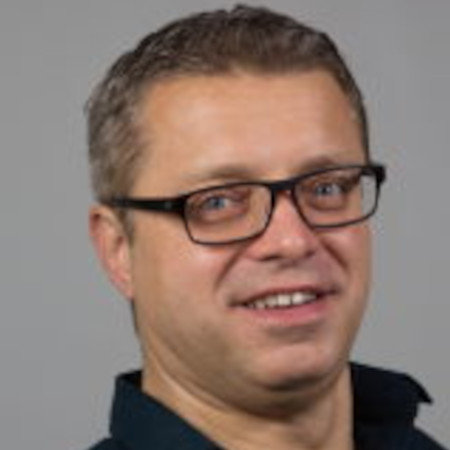Families of (strongly) dependent random variables, large deviation theory, Gaussian free fields, scaling limits, Wasserstein gradient flow, percolation models and statistical mechanics, high-diemsional probability theory, concentration inequality, random weak loop measures, renormalisation group theory, stochastic differential equations.
s dot adams at warwick dot ac dot uk

Tags
Mathematical Physics, Probability & Stochastic Analysis

### Ball, Keith

Professor Keith BallFunctional analysis, high-dimensional and discrete geometry, information theory and number theory.
K dot M dot Ball at warwick dot ac dot uk

Tags
Analysis, Combinatorics, Number Theory

### Barkley, Dwight

Professor Dwight Barkley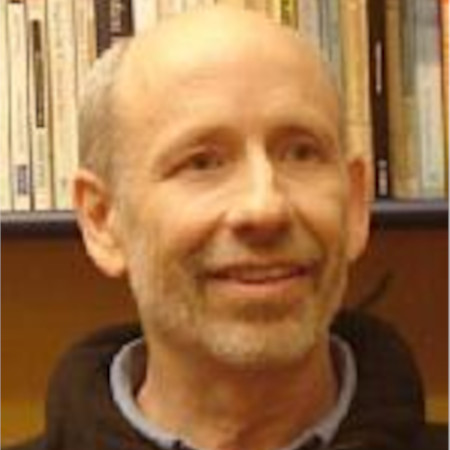Large-scale computation, mathematical modeling, hydrodynamic stability, bifurcation theory, transition to turbulence, reaction-diffusion equations, excitable media, percolation transitions.
d dot barkley at warwick dot ac dot uk

Tags
Computational Mathematics, Fluids & Continuum Mechanics, Modelling & Industrial Mathematics, Numerical Analysis, PDE Theory and Applications

### Bate, David

Dr David BateAnalysis, geometric measure theory, metric geometry.
David dot Bate at warwick dot ac dot uk

Tags
Analysis

### Bazzi, Marya

Dr Marya BazziMethods and algorithms for detecting and/or recovering structure in large time-dependent data and applying such tools to real world problems in online media, biology, digital humanities, and finance. Network science and in particular time-dependent networks, mesoscale structure, multilayer networks, stochastic block models, combinatorial optimization, and spectral optimization.
Marya dot Bazzi at warwick dot ac dot uk

Tags
Data Science, Graph Theory & Network Theory

### Böhning, Christian

Dr Christian Böhning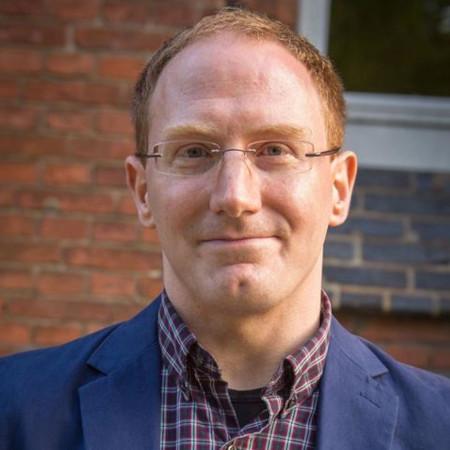Algebraic geometry, representation and invariant theory, derived category methods in birational geometry, birational automorphism groups, unramified cohomology and applications of K-theory in birational geometry.
C dot Boehning at warwick dot ac dot uk

Tags
Algebra, Algebraic Geometry

### Brambley, Ed

Dr Ed Brambley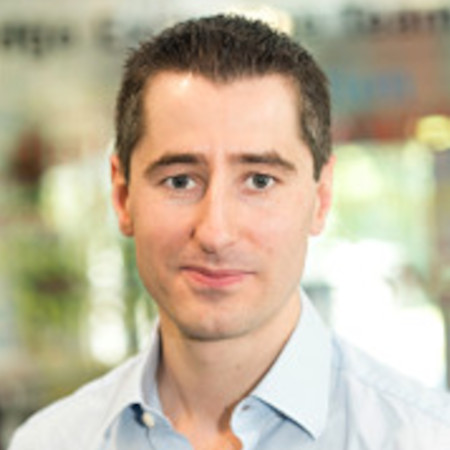Aeroacoustics (mathematical modelling and computational theory), continuum mechanics and plasticity (mathematical modelling of industrial metal forming processes), fluid dynamics, applied and industrial mathematical modelling.
E dot J dot Brambley at warwick dot ac dot uk

Tags
Fluids & Continuum Mechanics, Modelling & Industrial Mathematics

### Brown, Gavin

Professor Gavin Brown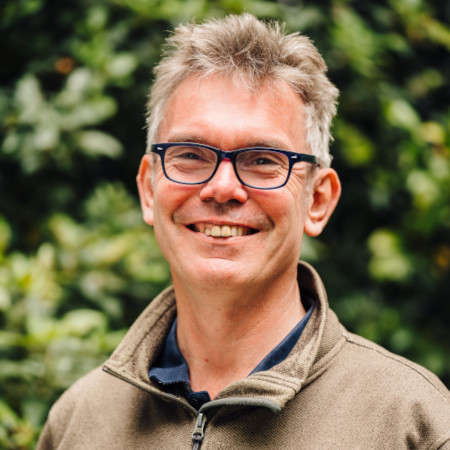Algebraic geometry, birational geometry and computational algebra.
G dot Brown at warwick dot ac dot uk

Tags
Algebraic Geometry, Computational Mathematics

### Burroughs, Nigel

Professor Nigel Burroughs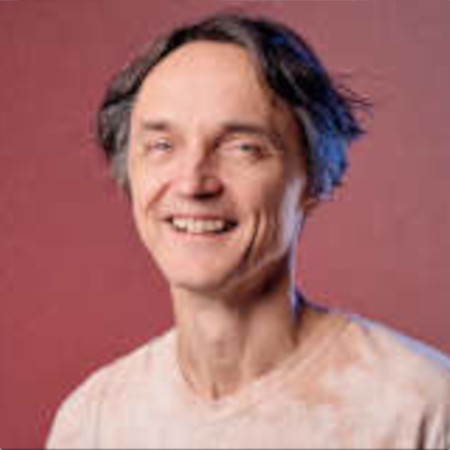Mathematical techniques applied to biological systems. Deterministic (ODEs, PDEs) and stochastic (SDEs, branching processes) models. Bayesian inference methods (MCMC) to infer parameters from experimental data. Model selection. Optimisation, including control theory (Pontryagin maximum principle, Hamilton-Jacobi-Bellman equations). Computational methods for spatial stochastic systems.
N dot J dot Burroughs at warwick dot ac dot uk

Tags
Computational Mathematics, Mathematical & Systems Biology

### Capdeboscq, Inna

Professor Inna Capdeboscq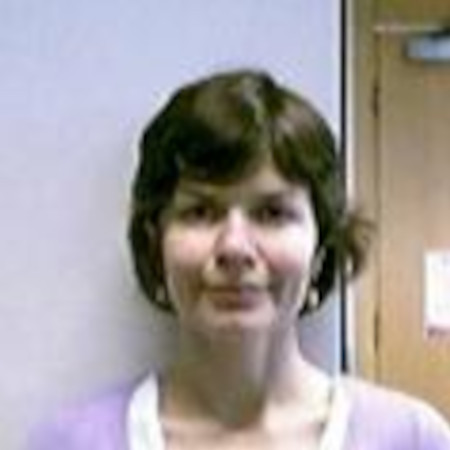Algebra, specifically group theory, both finite and infinite. The classification of finite simple groups.
I dot Capdeboscq at warwick dot ac dot uk

Tags
Algebra

### Chongchitnan, Siri

Dr Siri Chongchitnan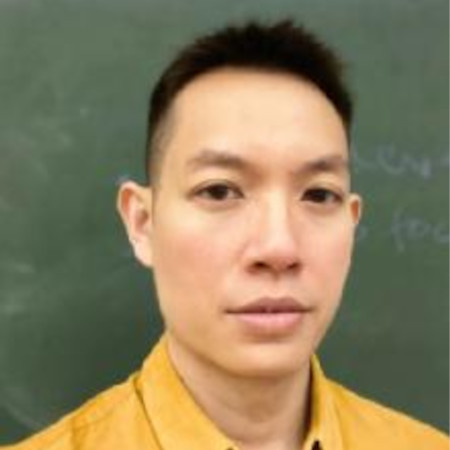Cosmology and theoretical astrophysics.
Siri dot Chongchitnan at warwick dot ac dot uk

Tags
Mathematical Physics

### Chow, Sam

Dr Sam Chow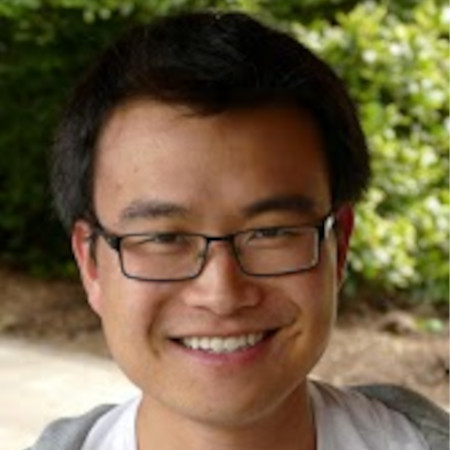Diophantine approximation, diophantine equations, arithmetic combinatorics, arithmetic statistics.
Sam dot Chow at warwick dot ac dot uk

Tags
Combinatorics, Number Theory

Dr Radu Cimpeanu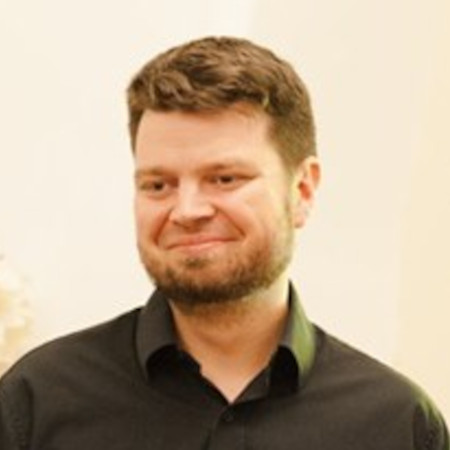Mathematical modelling and scientific computing. Fluid dynamics, acoustics, and multi-physics settings. Asymptotic and complex analysis, control theory, and hybrid methods built on data-driven approaches. Direct numerical simulation for interfacial flow problems involving drops, bubbles, and liquid films. Sustainable software and open science. Engagement with industrial partners.
Radu dot Cimpeanu at warwick dot ac dot uk

Tags
Computational Mathematics, Fluids & Continuum Mechanics, Modelling & Industrial Mathematics, Numerical Analysis, PDE Theory and Applications

### Dedner, Andreas

Dr Andreas Dedner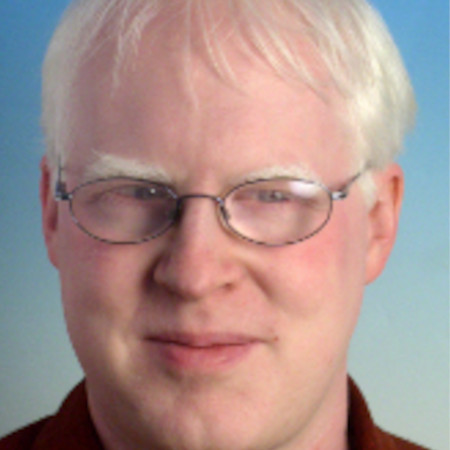Numerical analysis and scientific computing, higher order methods for solving non-linear evolution equations, generic software design for grid based numerical schemes, geophysical flows, radiation magnetohydrodynamics.
A dot S dot Dedner at warwick dot ac dot uk

Tags
Computational Mathematics, Fluids & Continuum Mechanics, Modelling & Industrial Mathematics, Numerical Analysis, PDE Theory and Applications

### Dotto, Emanuele

Dr Emanuele DottoAlgebraic topology, homotopy theory, algebraic K-theory, equivariant homotopy theory.
Emanuele dot Dotto at warwick dot ac dot uk

Tags
Algebra, Algebraic Topology

### During, Bertram

Professor Bertram DüringApplied and computational partial differential equations: modelling, analysis, numerical analysis, optimal control, applications in socio-economics and finance.
Bertram dot During at warwick dot ac dot uk

Tags
Computational Mathematics, Modelling & Industrial Mathematics, Numerical Analysis, PDE Theory and Applications

### Dyson, Louise

Dr Louise Dyson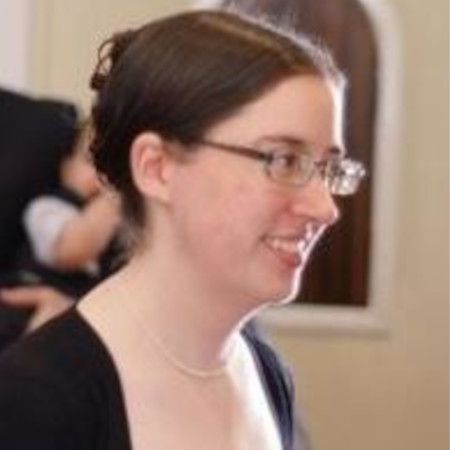Mathematical biology and infectious disease epidemiology including: neglected tropical diseases (NTDs); COVID-19; yaws; noise-induced bistable states; critical slowing down.
L dot Dyson at warwick dot ac dot uk

Tags
Epidemiology, Mathematical & Systems Biology, Modelling & Industrial Mathematics

### Elliott, Charles

Professor Charles Elliott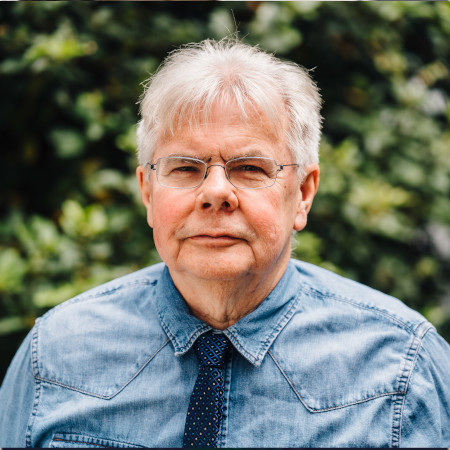Mathematical and numerical analysis of PDEs with applications in biology, material science, continuum mechanics and phase transitions. PDEs in complex and evolving domains including manifolds, finite element methods, free boundary problems, geometric evolution equations and surface growth, inverse problems, cell motility, biomembranes and PDE optimization.
C dot M dot Elliott at warwick dot ac dot uk

Tags
Computational Mathematics, Numerical Analysis, PDE Theory and Applications

Dr Adam EpsteinComplex analytic dynamics, Riemann surfaces, value-distribution theory.
A dot L dot Epstein at warwick dot ac dot uk

Tags
Dynamical Systems & Ergodic Theory, Geometry & Topology, Set Theory & Foundations

### Gallauer,Martin

Dr Martin Gallauer

Algebraic geometry & algebraic topology, motivic theory, tensor-triangular geometry, homotopy theory, rigid-analytic geometry, modular representation theory

martin dot gallauer at warwick dot ac dot uk

Tags
Algebra, Algebraic Geometry, Algebraic Topology

### Gelfreich, Vassili

Professor Vassili GelfreichAnalysis and dynamical systems.
V dot Gelfreich at warwick dot ac dot uk

Tags
Analysis, Dynamical Systems & Ergodic Theory

### Georgakopoulos, Agelos

Professor Agelos Georgakopoulos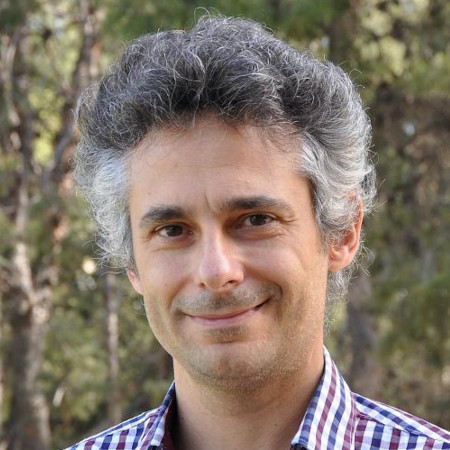Finite and infinite graphs, random walks and graphs, electrical networks, percolation theory, geometry and topology.
A dot Georgakopoulos at warwick dot ac dot uk

Tags
Combinatorics, Geometry & Topology, Graph Theory & Network Theory, Probability & Stochastic Analysis

### Gomes, Susana

Dr Susana Gomes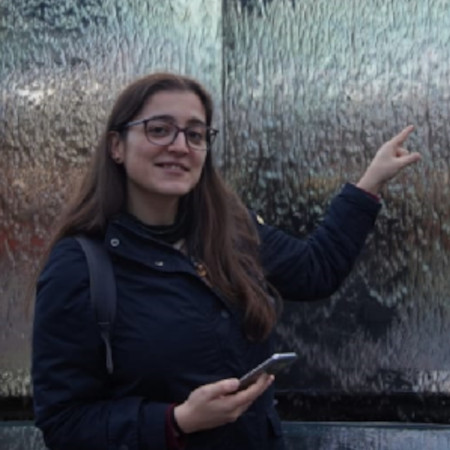Applied mathematics, modelling (PDEs and agent based models), control, and inverse problems. Applications to fluid dynamics and interfacial flows, pedestrian dynamics, and opinion dynamics.
Susana dot Gomes at warwick dot ac dot uk

Tags
Computational Mathematics, Fluids & Continuum Mechanics, Modelling & Industrial Mathematics, PDE Theory and Applications

### Grafka, Tobias

Dr Tobias Grafke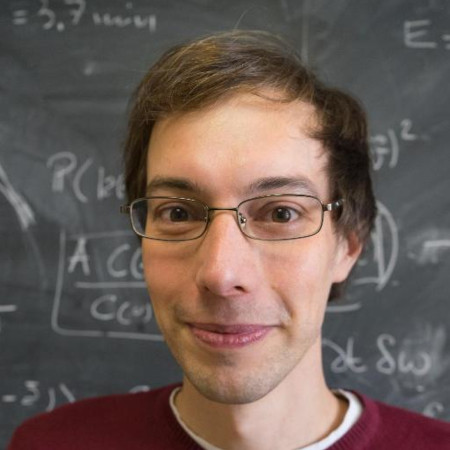Numerical and mathematical tools to analyse stochastic systems. Rare events, large deviation theory, metastability, non-equilibrium statistical mechanics, sampling algorithms. Applications include fluid dynamics and turbulence, atmosphere and ocean dynamics, climate, nonlinear waves, active matter, biological and chemical systems.
T dot Grafke at warwick dot ac dot uk

Tags
Computational Mathematics, Fluids & Continuum Mechanics, PDE Theory and Applications, Probability & Stochastic Analysis

### Greenlees, John

Professor John GreenleesAlgebraic topology, homotopy theory and commutative algebra, and especially equivariant cohomology theories and stable homotopy theory. Groups, symmetry, triangulated categories, derived categories, compact Lie groups, algebraic models.
John dot Greenlees at warwick dot ac dot uk

Tags
Algebra, Algebraic Geometry, Algebraic Topology, Geometry & Topology

Dr Adam Harper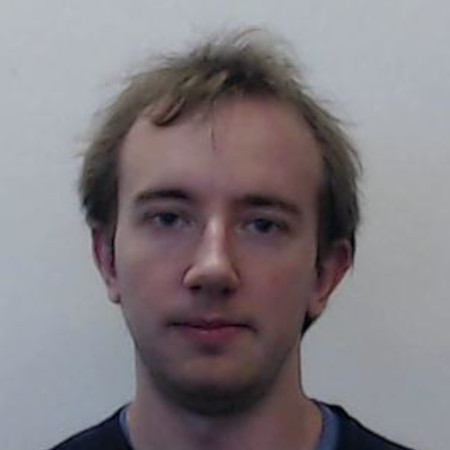Analytic number theory, and connections with probability and combinatorics.
A dot Harper at warwick dot ac dot uk

Tags
Analysis, Combinatorics, Number Theory, Probability & Stochastic Analysis

### Herzallah, Randa

Dr Randa HerzallahControl theory focusing primarily on developing reliable control strategies for many-body, multi-scale, stochastic systems exhibiting characteristics such as nonlinearity, uncertainty and hysteresis. Data analytics and machine learning. Systems modelling, signal processing, decentralized systems modelling and control, quantum systems modelling and control.
Randa dot Herzallah at warwick dot ac dot uk

Tags
Data Science, Graph Theory & Network Theory, Modelling & Industrial Mathematics, PDE Theory and Applications

### Hudson, Thomas

Dr Thomas HudsonMicromechanics, continuum mechanics, solid mechanics, crystalline defects, dislocations, plasticity, elasticity, asymptotic methods, gamma-convergence, homogenisation, coarse-graining, upscaling, discrete-to-continuum limits, stochastic modelling, large deviations principles, uncertainty quantification.
T dot Hudson dot 1 at warwick dot ac dot uk

Tags
Computational Mathematics, Fluids & Continuum Mechanics, Modelling & Industrial Mathematics, PDE Theory and Applications

### Ikenmeyer, Christian

Professor Christian IkenmeyerComputational complexity theory, tensor rank, representation theory, algebraic combinatorics, algebraic geometry.
Christian dot Ikenmeyer at warwick dot ac dot uk

Tags
Algebra, Algebraic Geometry, Combinatorics, Computational Mathematics

### Keeling, Matt

Professor Matt Keeling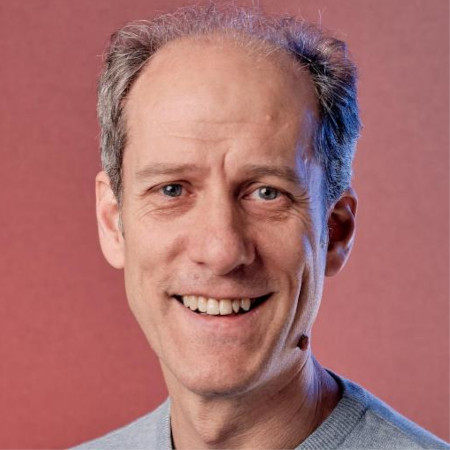Epidemiology, ecology, evolution, science for policy, networks, stochasticity, mathematical model, infection, disease, COVID-19.
M dot J dot Keeling at warwick dot ac dot uk

Tags
Epidemiology, Mathematical & Systems Biology

### Kirkilionis, Markus

Dr Markus Kirkilionis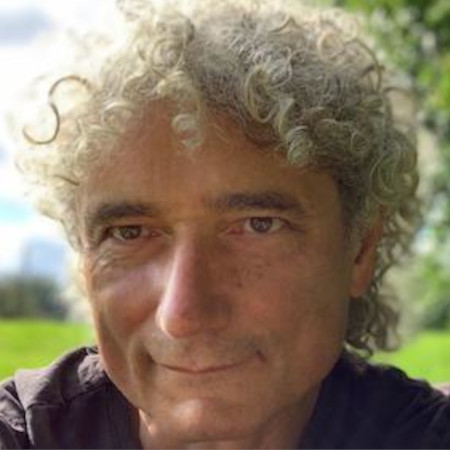Mathematical biology, dynamic network models, complex systems, numerical analysis, pattern formation, physiologically structured population models, (monotone) dynamical systems.
M dot A dot Kirkilionis at warwick dot ac dot uk

Tags
Dynamical Systems & Ergodic Theory, Graph Theory & Network Theory, Mathematical Physics, Mathematical & Systems Biology, Modelling & Industrial Mathematics

### Kropholler, Robert

Dr Robert KrophollerGeometric group theory, particularly in relation to Dehn functions, groups acting on cube complexes, finiteness properties and homological version of these.
Robert dot Kropholler at warwick dot ac dot uk

Tags
Geometry & Topology

### Li, Chunyi

Dr Chunyi Li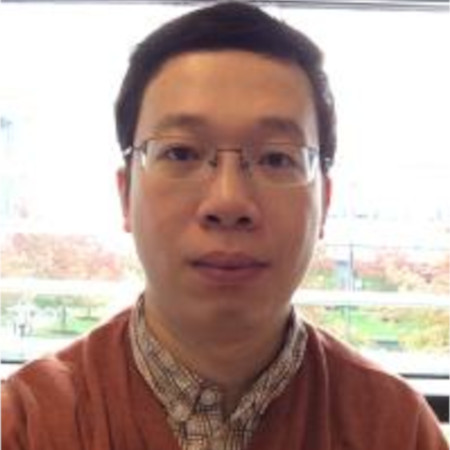Algebraic geometry, triangulated categories.
C dot Li dot 25 at warwick dot ac dot uk

Tags
Algebraic Geometry

### Loeffler, David

Professor David Loeffler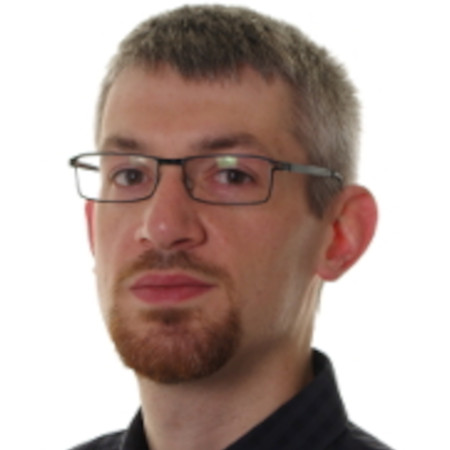Automorphic forms, special values of L-functions, Iwasawa theory.
D dot A dot Loeffler at warwick dot ac dot uk

Tags
Number Theory

### Lotz, Martin

Dr Martin Lotz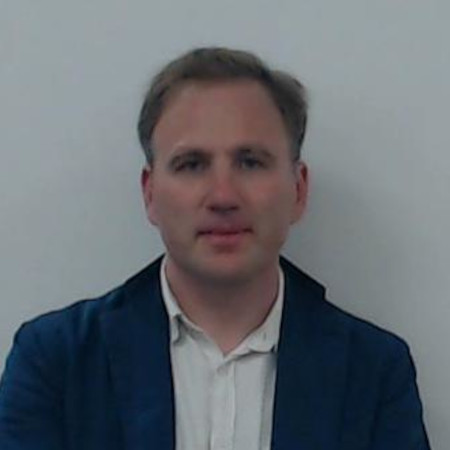Computational complexity, foundations of numerical analysis and optimization, integral geometry and geometric probability, computational algebraic geometry and topology.
Martin dot Lotz at warwick dot ac dot uk

Tags
Algebraic Geometry, Algebraic Topology, Combinatorics, Computational Mathematics, Data Science, Geometry & Topology, Numerical Analysis, Probability & Stochastic Analysis

Professor Vadim LozinGraph theory, Boolean functions, formal languages.
V dot Lozin at warwick dot ac dot uk

Tags
Combinatorics, Graph Theory & Network Theory

### MacKay, Robert

Professor Robert MacKayDynamical systems, network theory, plasma physics, economics, financial mathematics, spectral projections, general relativity, cosmology.
R dot S dot MacKay at warwick dot ac dot uk

Tags
Dynamical Systems & Ergodic Theory, Graph Theory & Network Theory, Mathematical Physics

### Maclagan, Diane

Professor Diane Maclagan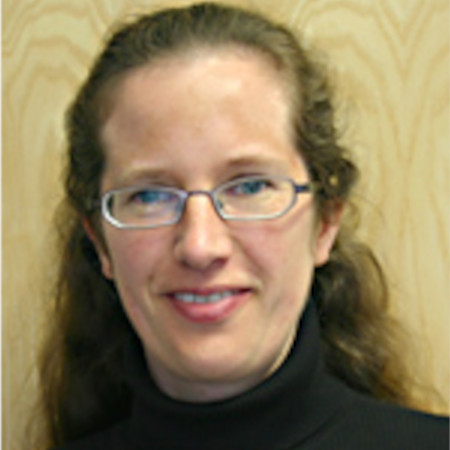Combinatorial and computational algebraic geometry and commutative algebra. Tropical geometry.
D dot Maclagan at warwick dot ac dot uk

Tags
Algebra, Algebraic Geometry, Combinatorics

### Máthé, András

Dr András Máthé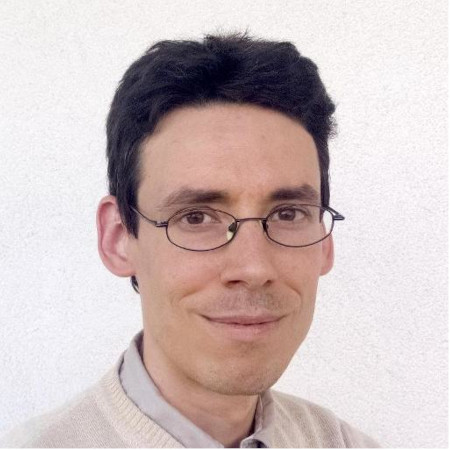Geometric measure theory, fractal geometry.
A dot Mathe at warwick dot ac dot uk

Tags
Analysis

### Melbourne, Ian

Professor Ian Melbourne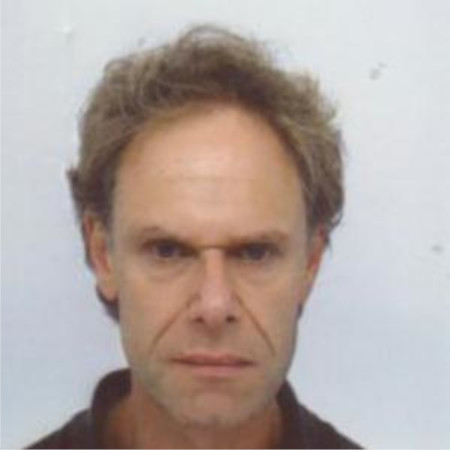Ergodic theory and dynamical systems. Links with probability theory and stochastic analysis.
I dot Melbourne at warwick dot ac dot uk

Tags
Dynamical Systems & Ergodic Theory, Probability & Stochastic Analysis

### Montenegro-Johnson, Tom

Professor Tom Montenegro-Johnson

Mathematical modelling, biological physics, soft and active matter, microbots and responsive materials, continuum mechanics, and the mathematics of touch

Tom dot Montenegro-Johnson at warwick dot ac dot uk

Tags
Computational Mathematics, Fluids & Continuum Mechanics, Modelling & Industrial Mathematics, PDE Theory and Applications

### Montgomery, Richard

Dr Richard Montgomery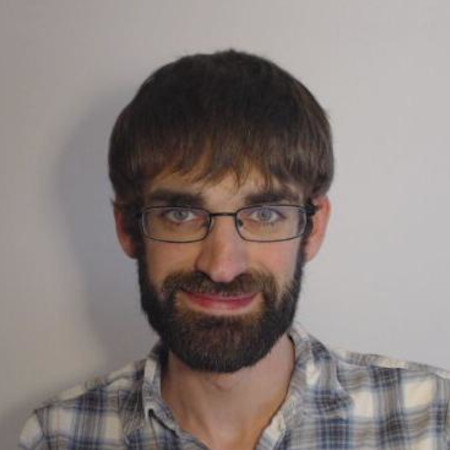Extremal and probabilistic combinatorics, and connections with other fields.
Richard dot Montgomery at warwick dot ac dot uk

Tags
Combinatorics

### Moreira, Joel

Dr Joel Moreira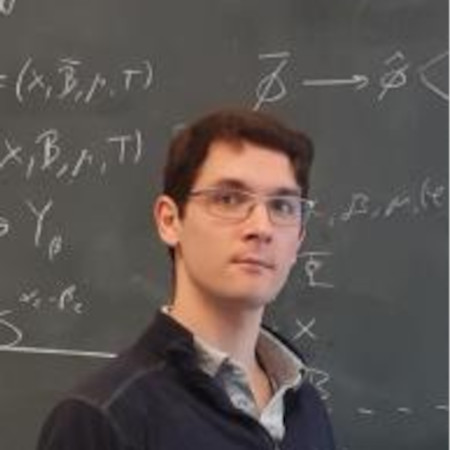Ergodic theory and topological dynamics, with emphasis on applications to Ramsey theory, additive combinatorics and number theory.
Joel dot Moreira at warwick dot ac dot uk

Tags
Combinatorics, Dynamical Systems & Ergodic Theory, Number Theory

### Pikhurko, Oleg

Professor Oleg Pikhurko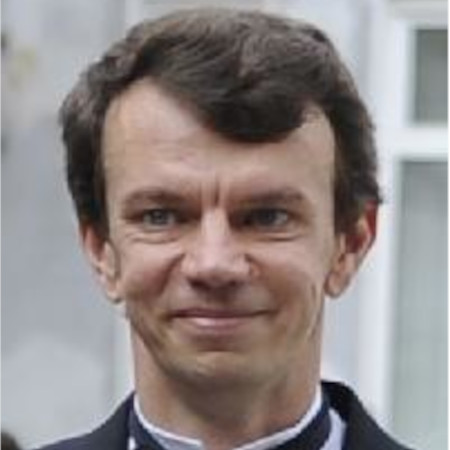Extermal and probabilistic combinatorics, graph theory, limits of discrete structures, descriptive combinatorics.
O dot Pikhurko at warwick dot ac dot uk

Tags
Combinatorics, Graph Theory & Network Theory, Set Theory & Foundations

### Planella, Ferran-Brosa

Dr Ferran Brosa Planella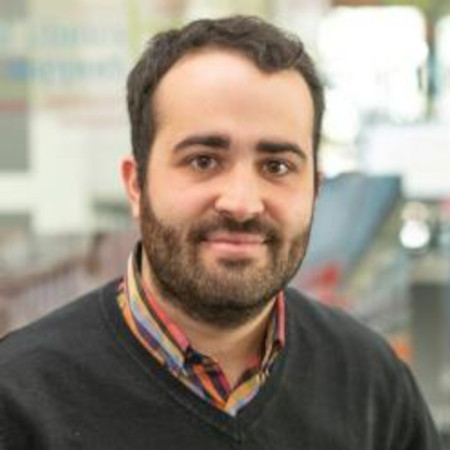Applied and industrial mathematics, mathematical modelling, asymptotic analysis, scientific computing, lithium-ion batteries, heat and mass transfer, continuum mechanics, moving boundary problems, dynamical systems.
Ferran dot Brosa-Planella at warwick dot ac dot uk

Tags
Computational Mathematics, Fluids & Continuum Mechanics, Modelling & Industrial Mathematics

### Pollicott, Mark

Professor Mark Pollicott
Dynamical systems, ergodic theory, Hausdorff dimension theory, thermodynamic formalism, hyperbolic geometry.
M dot Pollicott at warwick dot ac dot uk

Tags
Analysis, Dynamical Systems & Ergodic Theory

Dr Rohini Ramadas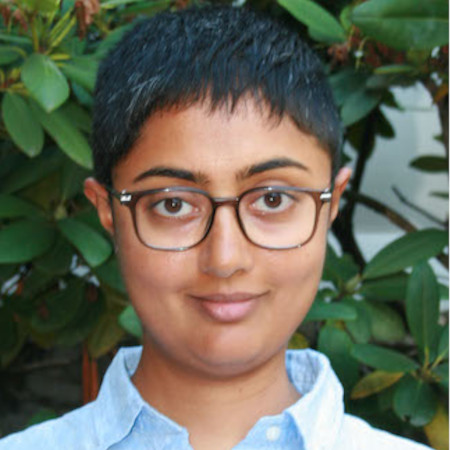Combinatorial algebraic geometry, complex dynamics, tropical geometry, moduli spaces.
Rohini dot Ramadas at warwick dot ac dot uk

Tags
Algebraic Geometry, Dynamical Systems & Ergodic Theory, Geometry & Topology

### Rand, David

Professor David RandSystems biology, especially applied to developmental biology, circadian rhythms and cancer. Mathematical results on global structure of bifurcations and catastrophes between Morse-Smale systems.
D dot A dot Rand at warwick dot ac dot uk

Tags
Dynamical Systems & Ergodic Theory, Mathematical & Systems Biology

### Reid, Miles

Professor Miles Reid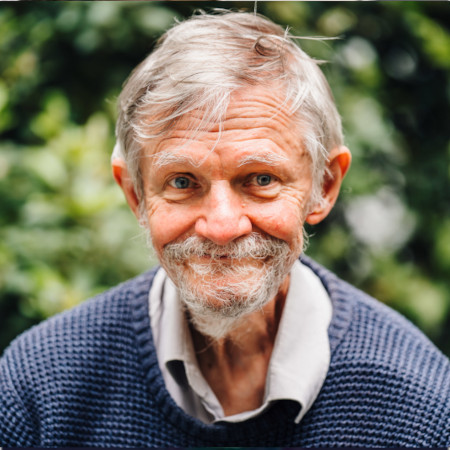Algebra and geometry, algebraic geometry, scheme theory, classification of varieties, graded rings, structure theory of Gorenstein rings, Gorenstein in small codimension, minimal models of 3-folds and higher dimensional algebraic varieties, singularities of 3-folds and higher dimensional algebraic varieties, group actions, group scheme actions, orbifolds and their resolution, McKay correspondence.
Miles dot Reid at warwick dot ac dot uk

Tags
Algebra, Algebraic Geometry

### Richardson, Magnus

Professor Magnus RichardsonMathematical and computational neuroscience involving experimental collaboration, data analysis, model building, theory of neuronal networks, and machine learning.
Magnus dot Richardson at warwick dot ac dot uk

Tags
Data Science, Graph Theory & Network Theory, Mathematical Physics, Mathematical & Systems Biology, Probability & Stochastic Analysis

### Rindler, Filip

Professor Filip RindlerGeometric measure theory, nonlinear PDEs, calculus of variations, mathematical material science.
F dot Rindler at warwick dot ac dot uk

Tags
Analysis, PDE Theory and Applications

### Robinson, James

Professor James RobinsonRigorous fluid dynamics, Navier-Stokes equations, infinite-dimensional dynamical systems, dimension theory, eigenfunction expansions.
J dot C dot Robinson at warwick dot ac dot uk

Tags
Analysis, Dynamical Systems & Ergodic Theory, Fluids & Continuum Mechanics, PDE Theory and Applications

### Rock, Kat

Professor Kat RockTransmission of vector-borne (insect-transmitted) infections, infections in low- or middle-income countries. Bringing together dynamic transmission modelling and health economic evaluation for real-world policy recommendations. ODEs, stochastics, optimisation, modelling for policy, and African sleeping sickness.
K dot S dot Rock at warwick dot ac dot uk

Tags
Epidemiology

### Rodrigo, José

Professor José RodrigoAnalysis, PDEs and theoretical fluid mechanics.
J dot Rodrigo at warwick dot ac dot uk

Tags
Analysis, Fluids & Continuum Mechanics, PDE Theory and Applications

### Rumynin, Dmitriy

Professor Dmitriy Rumynin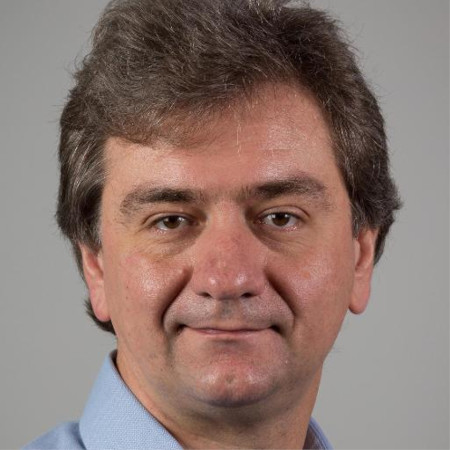Representation theory in the wide sense spreading into the following topics: algebraic geometry, algebraic groups, computer arithmetic, derived categories, hardware algorithms, homogeneous spaces, homotopy theory, Kac-Moody groups, Lie algebras, D-modules.
D dot Rumynin at warwick dot ac dot uk

Tags
Algebra, Algebraic Geometry, Algebraic Topology, Geometry & Topology, Number Theory

### Schleimer, Saul

Dr Saul Schleimer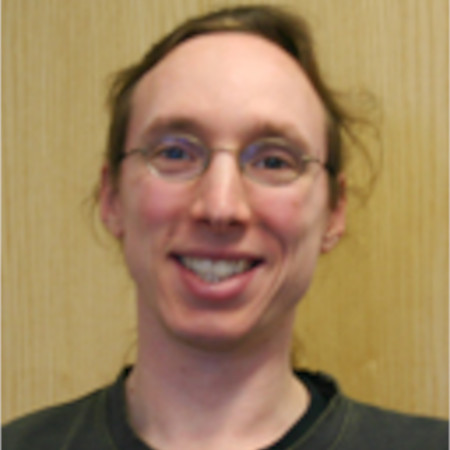Geometric topology, group theory, and computation.
S dot Schleimer at warwick dot ac dot uk

Tags
Dynamical Systems & Ergodic Theory, Geometry & Topology

### Schlichting, Marco

Dr Marco Schlichting
Algebraic K-theory and higher Grothendieck-Witt groups, homology and cohomology of classical groups, A^1-homotopy theory and motivic cohomology, derived categories, algebraic topology and algebraic geometry.
M dot Schlichting at warwick dot ac dot uk

Tags
Algebra, Algebraic Geometry, Algebraic Topology

### Schulze, Felix

Professor Felix Schulze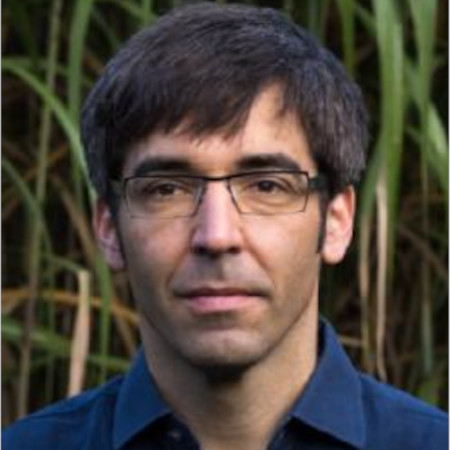Differential geometry, PDEs and geometric analysis, with particular interest in geometric flows (mean curvature flow, Ricci flow, Willmore flow), minimal surfaces and Willmore surfaces.
Felix dot Schulze at warwick dot ac dot uk

Tags
Analysis, Differential Geometry, PDE Theory and Applications

### Sharp, Richard

Professor Richard Sharp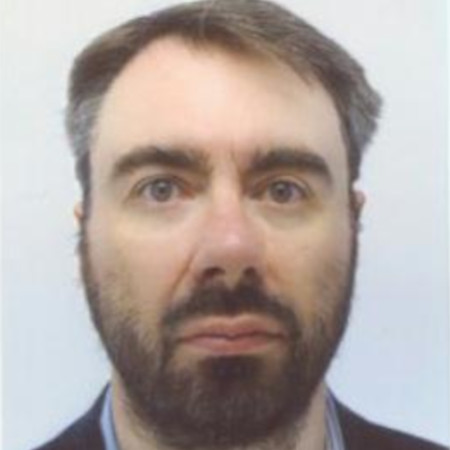Ergodic theory, dynamical systems, applications to geometry, combinatorial and geometric group theory, quantum chaos and noncommutative geometry.
R dot J dot Sharp at warwick dot ac dot uk

Tags
Dynamical Systems & Ergodic Theory

### Siksek, Samir

Professor Samir Siksek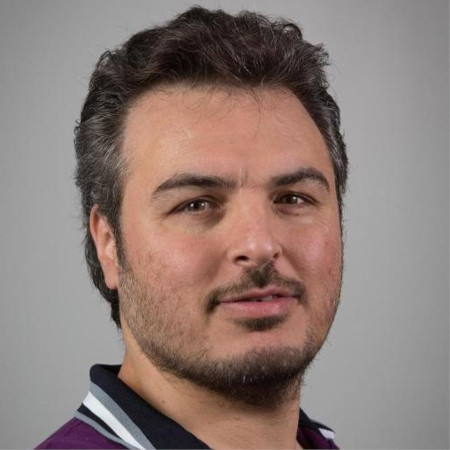Arithmetic of curves..
S dot Siksek at warwick dot ac dot uk

Tags
Number Theory

### Smillie, John

Professor John SmilliePolygonal billiards, flat surfaces, dynamics on moduli spaces, horocycle flows.
J dot Smillie at warwick dot ac dot uk

Tags
Dynamical Systems & Ergodic Theory

### Sohinger, Vedran

Dr Vedran SohingerNonlinear dispersive PDEs, harmonic analysis, and quantum many-body problems.
V dot Sohinger at warwick dot ac dot uk

Tags
Analysis, Mathematical Physics, PDE Theory and Applications

### Sprittles, James

Professor James SprittlesFluid dynamics, interfacial flows, computational modelling, micro/nanofluidics.
J dot E dot Sprittles at warwick dot ac dot uk

Tags
Computational Mathematics, Fluids & Continuum Mechanics, Modelling & Industrial Mathematics

### Stinner, Bjorn

Dr Björn Stinner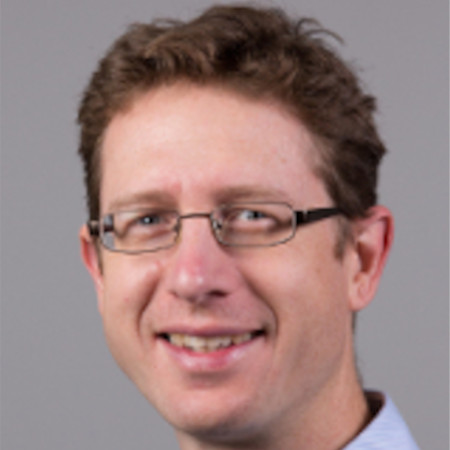Free and moving boundary problems, finite elements, phase field & diffuse interface modelling.
Bjorn dot Stinner at warwick dot ac dot uk

Tags
Computational Mathematics, Numerical Analysis, PDE Theory and Applications

### Sullivan, Tim

Dr Tim Sullivan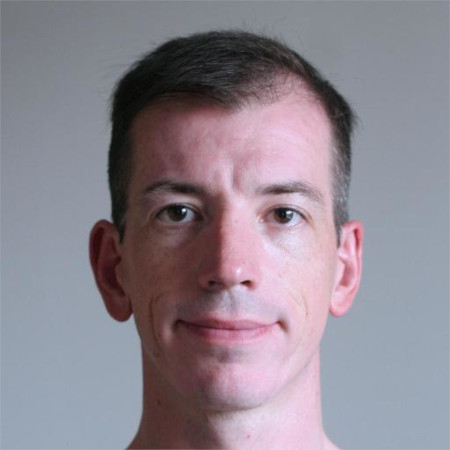Uncertainty quantification, numerical analysis, applied probability and statistics, and scientific computation. In particular: theoretical foundations of UQ, non-parametric Bayesian statistics, inverse problems in function spaces, optimisation-based methods and their relationship to Bayesian methods, methods for applied statistical problems, including dimension reduction and kernel-based machine learning, probabilistic perspectives on numerical methods, open-source software.
t dot j dot sullivan at warwick dot ac dot uk

Tags
Analysis, Computational Mathematics, Data Science, Numerical Analysis, PDE Theory and Applications, Probability & Stochastic Analysis

Dr Goncalo TabuadaAlgebraic topology, noncommutative algebraic geometry, K-theory, motives, homological & homotopical algebra.
Goncalo dot Tabuada at warwick dot ac dot uk

Tags
Algebra, Algebraic Geometry, Algebraic Topology

### Testa, Damiano

Dr Damiano Testa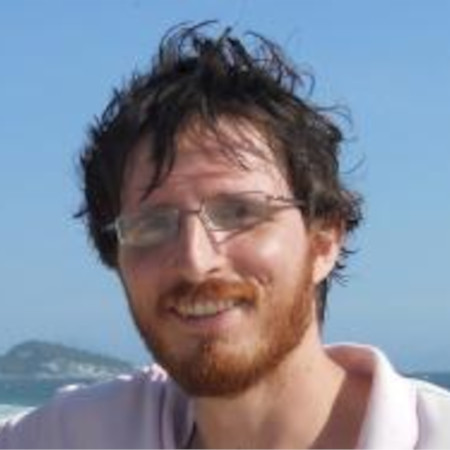Algebraic geometry and interactions with number theory. Formalization of mathematics.
D dot Testa at warwick dot ac dot uk

Tags
Algebraic Geometry, Number Theory

### Theil, Florian

Dr Florian Theil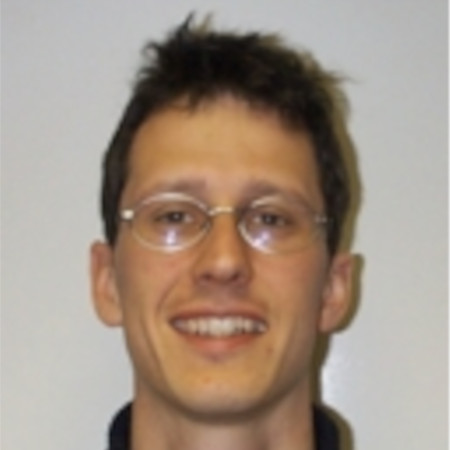Analysis of particle systems. Modelling of Li-ion batteries.
F dot Theil at warwick dot ac dot uk

Tags
Mathematical Physics, Modelling & Industrial Mathematics, PDE Theory and Applications

Dr Adam Thomas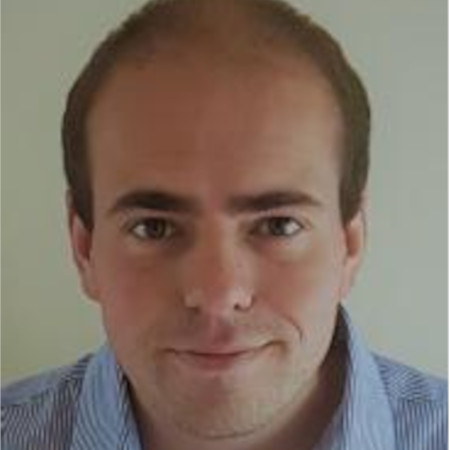Algebraic groups, Serre's notion of complete reducibility, Lie algebras, finite groups of Lie type, permutation groups, representation theory.
Adam dot R dot Thomas at warwick dot ac dot uk

Tags
Algebra

### Tildesley, Michael

Professor Michael TildesleyMathematical modelling of infectious diseases including avian influenza, foot-and-mouth disease, rabies and bovine tuberculosis. Modelling of control policies in the presence of partial information.
M dot J dot Tildesley at warwick dot ac dot uk

Tags
Computational Mathematics, Epidemiology, Mathematical & Systems Biology

### Topping, Peter

Professor Peter ToppingGeometric analysis, PDE theory, differential geometry, geometric flows, Ricci flow.
P dot M dot Topping at warwick dot ac dot uk

Tags
Analysis, Differential Geometry, Geometry & Topology, PDE Theory and Applications

### Tracey, Gareth

Dr Gareth TraceyFinite group theory, particularly: generation properties of finite groups, properties of almost simple groups, permutation groups and associated combinatorics, and probabilistic group theory.
Gareth dot Tracey at warwick dot ac dot uk

Tags
Algebra

### Tribe, Roger

Dr Roger TribeTopics in probability theory. stochastic processes, particle systems, PDEs, random matrices.
R dot P dot Tribe at warwick dot ac dot uk

Tags
Analysis, Mathematical Physics, Probability & Stochastic Analysis

### Ueltschi, Daniel

Professor Daniel Ueltschi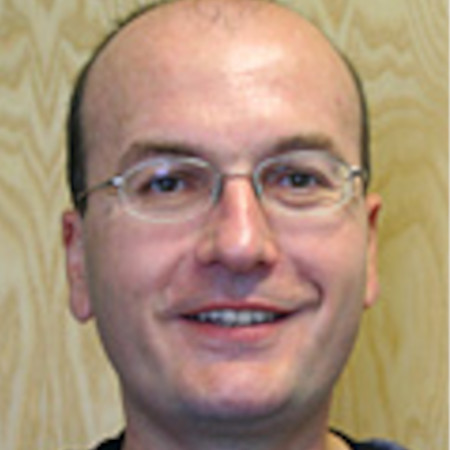Classical and quantum statistical mechanic, phase transitions, classical and quantum spin systems.
D dot Ueltschi at warwick dot ac dot uk

Tags
Mathematical Physics, Probability & Stochastic Analysis

### Vogtmann, Karen

Professor Karen Vogtmann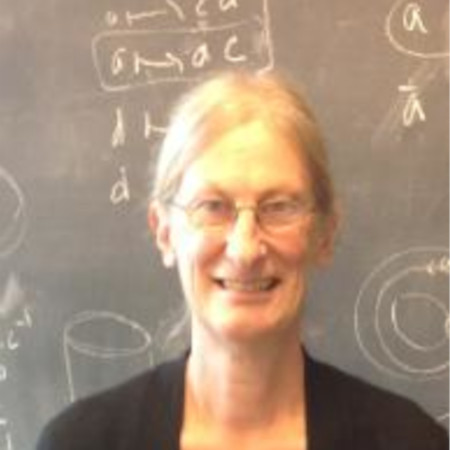Moduli spaces of graphs and CAT(0) structures, and connections of these with other areas of mathematics, physics and biology.
K dot Vogtmann at warwick dot ac dot uk

Tags
Geometry & Topology

### Wolfram, Marie-Therese

Professor Marie-Therese Wolfram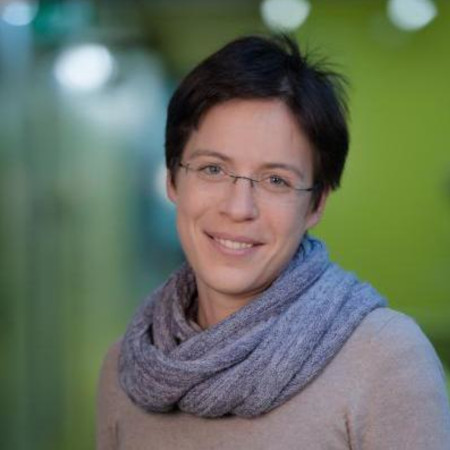Applied PDEs, inverse problems, mathematical modeling.
M dot Wolfram at warwick dot ac dot uk

Tags
Modelling & Industrial Mathematics, PDE Theory and Applications

### Wood, David

Dr David Wood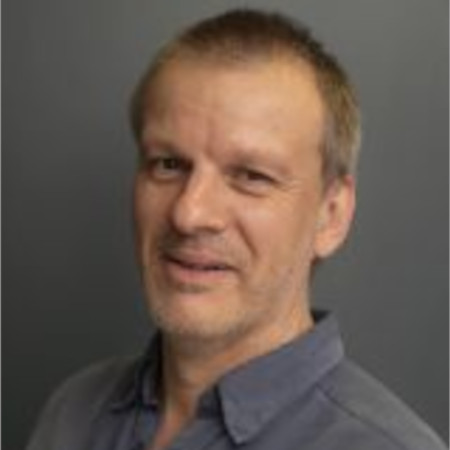Dynamical systems, bifurcations with symmetry, applications to biology and industry.
David dot Wood at warwick dot ac dot uk

Tags
Dynamical Systems & Ergodic Theory, Mathematical & Systems Biology, Modelling & Industrial Mathematics

### Zaboronski, Oleg

Professor Oleg Zaboronski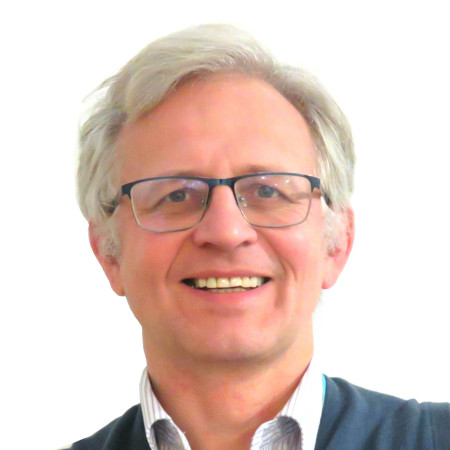Non-equilibrium statistical mechanics of interacting particle systems, random matrices and integrable systems.
mascy at warwick dot ac dot uk

Tags
Mathematical Physics, Probability & Stochastic Analysis

### Zhang, Weiyi

Dr Weiyi Zhang
Symplectic topology, complex geometry and their interactions.
Weiyi dot Zhang at warwick dot ac dot uk

Tags
Geometry & Topology

### Zygouras, Nikolaos

Professor Nikolaos Zygouras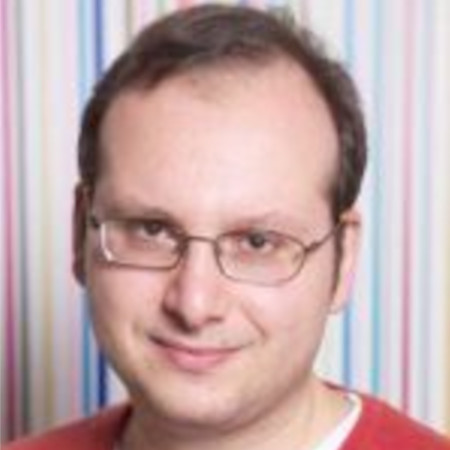Probability theory and its relations to mathematical physics and statistical mechanics.
N dot Zygouras at warwick dot ac dot uk

Tags
Mathematical Physics, Probability & Stochastic Analysis